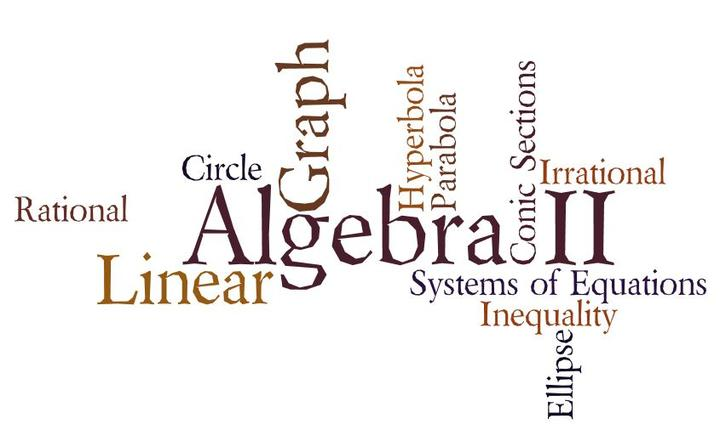# COURSE DESCRIPTIONAlgebra II expands the learning of foundational topics covered in Algebra, giving students a more complex understanding of the subject matter. The curriculum includes working with polynomial expressions and solving equations, including rational expressions. Concepts such as radicals, complex numbers, logarithms, and sequence and series are explained. The course concludes with the fundamentals of trigonometry including the unit circle, trigonometric graphs and translations, solving trigonometric equations and proving trigonometric identities as well as applications of trigonometry. Lessons are designed using a best practice model that includes scaffolding, vocabulary, prior knowledge, guided practice, independent practice, and written response opportunities for students to solve, analyze, and evaluate concepts. The lessons are designed according to the New York State Learning Standards.

# CHAPTERS

1A – Polynomials – From Base Ten to Base X
• Algebraic Patterns
• Multiplying Polynomials
• Dividing Polynomials
• Polynomial Identities
• Pythagorean Triples

1B – Factoring – Its Use and Its Obstacles
• Review: Factor Quadratics to Find Solutions and Zeros
• Factoring Quadratic Expressions When the Leading Coefficient is Greater Than One
• Factoring Fourth Degree Polynomials
• Factoring Polynomials – Other Strategies
• Factoring Third Degree Polynomials
• Mastering Factoring – Extending Strategies
• Analyzing the Graphs of Polynomial Functions
• The Remainder Theorem
• Solve Problems Using Polynomial Functions

1C – Solving and Applying Equations – Polynomial, Rational, and Radical
• Rational Polynomial Expressions
• Rational Polynomial Expressions – Add, Subtract, Multiply and Divide
• Solving Rational Polynomial Equations
• Solve Linear Systems in Three Variables
• Graphing Systems of Equations
• Focus-Directrix Definition of a Parabola
• Congruence and Similarity of Parabolas

1D – Complex Numbers Overcome All Obstacles
• Properties of Complex Numbers
• Solving Polynomial Equations with Complex Solutions
• The Fundamental Theorem of Algebra

2A – Exponents and Logarithms
• Simplify Algebraic Expressions with Negative and Fractional Exponents
• Rewriting Algebraic Expressions Involving Radicals as Fractional Exponents
• Evaluate Numerical Expressions with Fractional or Negative Exponents
• Graphing Exponential & Logarithmic Functions
• Solving Exponential Equations
• Evaluate Logrithmic Expressions in Any Base
• Properties of Logarithms: Rewriting Logarithm Expressions as One Equivalent Log
• Properties of Logarithms: Rewriting Single Logarithm Expressions as Multiple Logarithms
• Solving Equations Involving Logarithms
• Applications of Exponential Functions

2B – Series and Sequences
• Arithmetic Sequences
• Geometric Sequences
• Mathematical Patterns
• Sigma Notation & Summation of a Series
• Binomial Expansion

3A – Statistics
• Gathering Data
• Types of Bias in Surveys
• Organizing Data
• Measures of Central Tendency
• Measures of Dispersion: Interquartile Range
• Variance and Standard Deviation Including the Normal Curve
• Bivariate Statistics and Linear Regression
• Non-Linear Regression

3B – Probability
• Introduction to Permutations and Combinations
• Permutations
• Combinations
• Theoretical Probability
• Sample Spaces
• Binomial Probability Formula
• Using the Normal Distribution to Approximate Binomial Probabilities